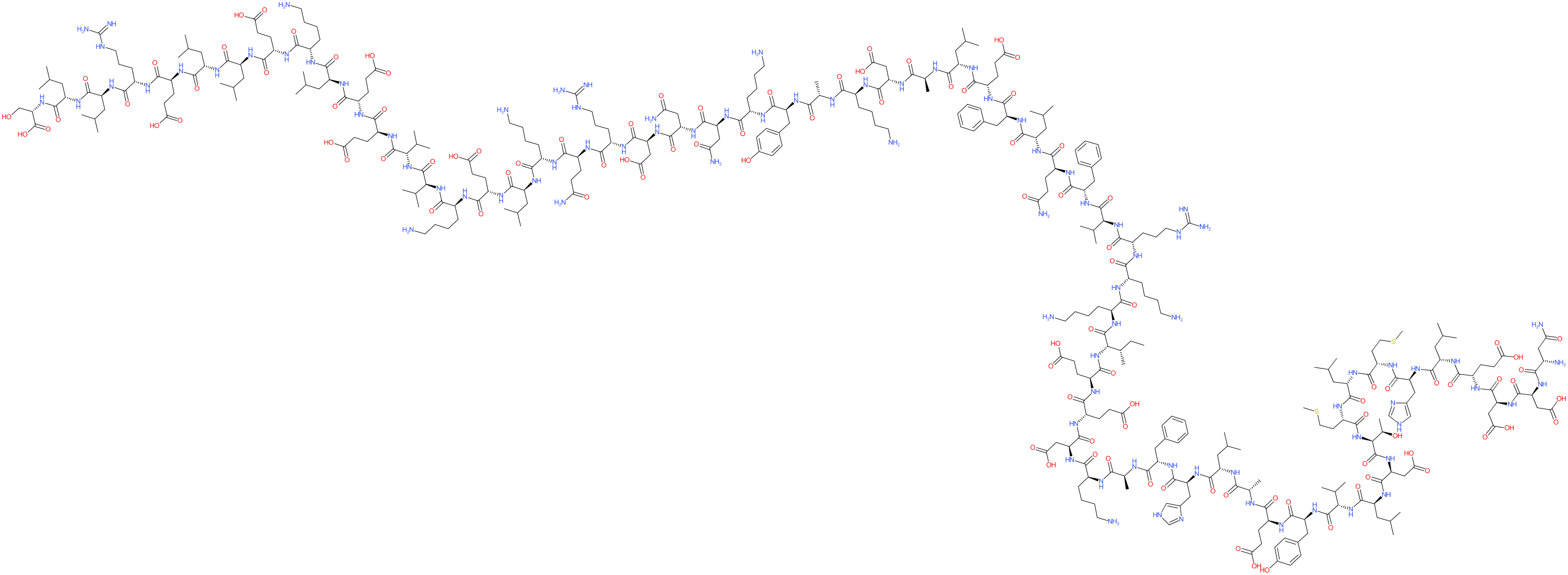#### GtoPdb Ligand ID: 11237

Comment: A synthetic peptide that binds potently to the ACE2 binding domain of the SARS-CoV-2 spike protein, and blocks entry of SARS-CoV-2 in vitro . The chemical SMILES was generated using the HELM editor. The crystal structure of LCB3 in complex with SARS-CoV-2 spike protein has been deposited with the PDB, ID 7JZN2D StructureSMILES / InChI / InChIKeyCanonical SMILES NCCCC[C@@H](C(=O)N[C@H](C(=O)N[C@H](C(=O)N[C@H](C(=O)N[C@H](C(=O)N[C@H](C(=O)N[C@H](C(=O)N[C@H](C(=O)N[C@H](C(=O)N[C@H](C(=O)N[C@H](C(=O)N[C@H](C(=O)N[C@H](C(=O)N[C@H](C(=O)N[C@H](C(=O)N[C@H](C(=O)N[C@H](C(=O)N[C@H](C(=O)N[C@H](C(=O)N[C@H](C(=O)N[C@H](C(=O)N[C@H](C(=O)N[C@H](C(=O)N[C@H](C(=O)N[C@H](C(=O)N[C@H](C(=O)N[C@H](C(=O)N[C@H](C(=O)N[C@H](C(=O)N[C@H](C(=O)N[C@H](C(=O)N[C@H](C(=O)N[C@H](C(=O)N[C@H](C(=O)N[C@H](C(=O)N[C@H](C(=O)N[C@H](C(=O)N[C@H](C(=O)O)CO)CC(C)C)CC(C)C)CCCNC(=N)N)CCC(=O)O)CC(C)C)CC(C)C)CCC(=O)O)CCCCN)CC(C)C)CCC(=O)O)CCC(=O)O)C(C)C)C(C)C)CCCCN)CCC(=O)O)CC(C)C)CCCCN)CCC(=O)N)CCCNC(=N)N)CC(=O)O)CC(=O)N)CC(=O)N)CCCCN)Cc1ccc(cc1)O)C)CCCCN)CC(=O)O)C)CC(C)C)CCC(=O)O)Cc1ccccc1)CC(C)C)CCC(=O)N)Cc1ccccc1)C(C)C)CCCNC(=N)N)NC(=O)[C@@H](NC(=O)[C@H]([C@H](CC)C)NC(=O)[C@@H](NC(=O)[C@@H](NC(=O)[C@@H](NC(=O)[C@@H](NC(=O)[C@@H](NC(=O)[C@@H](NC(=O)[C@@H](NC(=O)[C@@H](NC(=O)[C@@H](NC(=O)[C@@H](NC(=O)[C@@H](NC(=O)[C@H](C(C)C)NC(=O)[C@@H](NC(=O)[C@@H](NC(=O)[C@H]([C@H](O)C)NC(=O)[C@@H](NC(=O)[C@@H](NC(=O)[C@@H](NC(=O)[C@@H](NC(=O)[C@@H](NC(=O)[C@@H](NC(=O)[C@@H](NC(=O)[C@@H](NC(=O)[C@H](CC(=O)N)N)CC(=O)O)CC(=O)O)CCC(=O)O)CC(C)C)Cc1nc[nH]c1)CCSC)CC(C)C)CCSC)CC(=O)O)CC(C)C)Cc1ccc(cc1)O)CCC(=O)O)C)CC(C)C)Cc1nc[nH]c1)Cc1ccccc1)C)CCCCN)CC(=O)O)CCC(=O)O)CCC(=O)O)CCCCN Isomeric SMILES NCCCC[C@@H](C(=O)N[C@H](C(=O)N[C@H](C(=O)N[C@H](C(=O)N[C@H](C(=O)N[C@H](C(=O)N[C@H](C(=O)N[C@H](C(=O)N[C@H](C(=O)N[C@H](C(=O)N[C@H](C(=O)N[C@H](C(=O)N[C@H](C(=O)N[C@H](C(=O)N[C@H](C(=O)N[C@H](C(=O)N[C@H](C(=O)N[C@H](C(=O)N[C@H](C(=O)N[C@H](C(=O)N[C@H](C(=O)N[C@H](C(=O)N[C@H](C(=O)N[C@H](C(=O)N[C@H](C(=O)N[C@H](C(=O)N[C@H](C(=O)N[C@H](C(=O)N[C@H](C(=O)N[C@H](C(=O)N[C@H](C(=O)N[C@H](C(=O)N[C@H](C(=O)N[C@H](C(=O)N[C@H](C(=O)N[C@H](C(=O)N[C@H](C(=O)N[C@H](C(=O)O)CO)CC(C)C)CC(C)C)CCCNC(=N)N)CCC(=O)O)CC(C)C)CC(C)C)CCC(=O)O)CCCCN)CC(C)C)CCC(=O)O)CCC(=O)O)C(C)C)C(C)C)CCCCN)CCC(=O)O)CC(C)C)CCCCN)CCC(=O)N)CCCNC(=N)N)CC(=O)O)CC(=O)N)CC(=O)N)CCCCN)Cc1ccc(cc1)O)C)CCCCN)CC(=O)O)C)CC(C)C)CCC(=O)O)Cc1ccccc1)CC(C)C)CCC(=O)N)Cc1ccccc1)C(C)C)CCCNC(=N)N)NC(=O)[C@@H](NC(=O)[C@H]([C@H](CC)C)NC(=O)[C@@H](NC(=O)[C@@H](NC(=O)[C@@H](NC(=O)[C@@H](NC(=O)[C@@H](NC(=O)[C@@H](NC(=O)[C@@H](NC(=O)[C@@H](NC(=O)[C@@H](NC(=O)[C@@H](NC(=O)[C@@H](NC(=O)[C@H](C(C)C)NC(=O)[C@@H](NC(=O)[C@@H](NC(=O)[C@H]([C@H](O)C)NC(=O)[C@@H](NC(=O)[C@@H](NC(=O)[C@@H](NC(=O)[C@@H](NC(=O)[C@@H](NC(=O)[C@@H](NC(=O)[C@@H](NC(=O)[C@@H](NC(=O)[C@H](CC(=O)N)N)CC(=O)O)CC(=O)O)CCC(=O)O)CC(C)C)Cc1nc[nH]c1)CCSC)CC(C)C)CCSC)CC(=O)O)CC(C)C)Cc1ccc(cc1)O)CCC(=O)O)C)CC(C)C)Cc1nc[nH]c1)Cc1ccccc1)C)CCCCN)CC(=O)O)CCC(=O)O)CCC(=O)O)CCCCN InChI Key AYKCULXVFGARTK-NUIKKLCPSA-N
 ClassificationCompound class Peptide or derivative
 Database LinksGtoPdb PubChem SID 434122297 Search Google for chemical match using the InChIKey AYKCULXVFGARTK-NUIKKLCPSA-N Search Google for chemicals with the same backbone AYKCULXVFGARTK UniChem Compound Search for chemical match using the InChIKey AYKCULXVFGARTK-NUIKKLCPSA-N UniChem Connectivity Search for chemical match using the InChIKey AYKCULXVFGARTK-NUIKKLCPSA-N